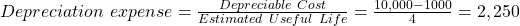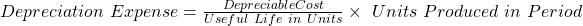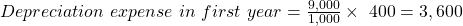Long Lived Assets | IFT World
IFT Notes for Level I CFA® Program
IFT Notes for Level I CFA® Program

# Part 3

## 6. Depreciation of Long-Lived Assets: Methods and Calculation

Under the cost model of reporting long-lived assets, the capitalized cost of a tangible (intangible) long-lived asset is expensed through a process called depreciation (amortization).

### 6.1 Depreciation Methods and Calculation of Depreciation Expense

Depreciation methods are:

• Straight line – The cost of an asset is evenly distributed over the asset’s useful life.
• Accelerated methods – A higher depreciation expense is recorded in the early years and lower depreciation expense is recorded in the later years of an asset’s life.
• Units of production – Cost allocated is based on the actual use of an asset in a particular period.

Formulae

Straight line depreciation expense = depreciable cost / estimated useful life

DDB depreciation expense = 2 x straight-line rate x beginning book value

Units of Production depreciation expense per unit = depreciable cost/useful life in units

Carrying amount = historical cost – accumulated depreciation
Depreciable cost = historical cost – estimated residual value

Example

Consider three companies with names based on their depreciation method:

1. Straight Line (SL) Inc.
2. Double Declining Balance (DDB) Inc.
3. Units of Production (UOP) Inc.

Each company purchases identical equipment for 10,000 and makes similar assumptions; estimated useful life = 4 years; residual value = 1,000; productive capacity = 1,000 units. Production over 4 years is 400, 300, 200, and 100 respectively. Complete the table below for each company.

 Beginning Net Book Value Depreciation Expense Accumulated Depreciation Ending Net Book Value Year 1 Year 2 Year 3 Year 4

Solution:

Straight-Line Method:Straight-line Method Beginning Net Book Value Depreciation Expense Accumulated Depreciation Ending Net Book Value Year 1 10,000 2,250 2,250 7,750 Year 2 7,750 2,250 4,500 5,500 Year 3 5,500 2,250 6,750 3,250 Year 4 3,250 2,250 9,000 1,000

Double-Declining Method (DDM)

The rate of depreciation in double-declining method (DDM) is twice that of straight-line method. It is 25% for straight-line method (100% in 4 years = 25%). The rate of decline for DDM is 50%. Depreciation expense for first year = 0.25 x 2 x 10,000 = 5,000 and so on for the subsequent years. Once the net book value is equal to the residual value there is no further depreciation.

 Double-declining balance method Beginning Net Book Value Depreciation Expense Accumulated Depreciation Ending Net Book Value Year 1 10,000 5,000 5,000 5,000 Year 2 5,000 2,500 7,500 2,500 Year 3 2,500 1,250 8,750 1,250 Year 4 1,250 250 9,000 1,000

Units of Production Method:Note that we use the depreciable cost of 9,000 which is the original cost (10,000) minus the residual value (1,000).

 Units of production method Beginning Net Book Value Depreciation Expense Accumulated Depreciation Ending Net Book Value Year 1 10,000 3,600 3,600 6,400 Year 2 6,400 2,700 6,300 3,700 Year 3 3700 1,800 8,100 1,900 Year 4 1900 900 9,000 1,000

Some points to be noted:

• The beginning book value is the same for all the three methods.
• The total depreciation over 4 years for all three companies (or under three depreciation methods) should be the same.

Impact of Depreciation Methods on Financial Statements

The choice of depreciation method affects the amounts reported for assets, operating and net income, which in turn, affect the financial ratios. The relationships indicated in the table below are for the early years of an asset’s life.

 Straight Line (SL) Accelerated (DDB) Interpretation Depreciation Expense Lower Higher Compared to SL, the rate of depreciation for DDB is double, making the depreciation expense higher in the initial years. Net Income Higher Lower Compared to SL, depreciation expense is higher in initial years for DDB making net income lower. Assets Higher Lower Compared to SL, depreciation and accumulated depreciation are higher for DDB making the net book value of assets lower in the initial years. Equity Higher Lower Equity = assets – liabilities. Liabilities are not affected. Since assets are lower for DDB, equity is also lower. Return on Assets (NI/Assets) Higher Lower Compared to SL, ROA for DDB is lower in earlier years because percentage impact on the numerator (net income) is more than the percentage impact on the denominator (assets).  Percentage impact on assets is lower because equipment is generally a small percentage of assets. Return on Equity Higher Lower Compared to SL, ROE for DDB is lower in earlier years because percentage impact on net income is more than the percentage impact on equity. Asset Turnover Lower Higher Revenue is not impacted by the choice of depreciation method. Net book value of assets is lower for the DDB method making asset turnover higher. Operating Profit Margin Higher Lower Revenue is not impacted. EBIT is lower for DDB in the initial years which makes operating profit margin lower.

The above relationships are for the initial years of an asset. These reverse in the later years if the firm’s capital expenditure declines.

Component Method of Depreciation

In this method, individual components or parts of an asset are depreciated separately at different rates. For example, in an aircraft, it may be prudent to depreciate engine, frame and interior furnishings separately.

• IFRS requires companies to use the component method of depreciation i.e. depreciate each component separately.
• US GAAP allows component depreciation but the method is often not used in practice.

## 7. Amortization of Long-Lived Assets: Methods and Calculation

Amortization is similar in concept to depreciation. The term amortization applies to intangible assets, and term depreciation applies to tangible assets.
Amortization methods for intangible assets with finite lives are the same as those used in depreciation:

• Straight-line
• Accelerated
• Units-of-production

The calculation of amortization expense is also similar to that of depreciation expense (covered earlier).

Zoom Classes for Nov-23 Exam Starting Now!
This is default text for notification bar